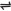## Production of Hydrogen

Commercial Production of Hydrogen

Hydrogen can be produced commercially through the following methods:

(1). From Water - Gas (this is a mixture of carbon(II) oxide and hydrogen). Water gas can be produced from:

(a). Hydrocarbons, e.g. Methane, CH4 - when methane is passed into steam, over nickel catalyst at - 800oC and 30 atm, water gas is produced.

CH4(g) + H2O(g) → CO(g) + 3H2(g)

(b). From the passage of steam through a mass of hot coke at a very high temperature (about 1000oC).

C(s) + H2O(g) → CO(g) + H2(g)

The water gas is mixed with more steam and passed over iron(III) oxide (as catalyst) at 450oC. More hydrogen is produced and carbon(II) oxide gets oxidized to carbondioxide. I.e.

CO(g) + 3H2(g) + H2O(g) → CO2(g) + 4H2(g)

The carbondioxide is removed by dissolving the above products in water under 30 atm pressure - CO2 dissolves.

H2O(g) + CO2(g) → H2CO3(aq)

The gas is then passed through copper(I) methanoate in ammonia solution under pressure to absorb any trace of carbon(II) oxide which might be present as impurity if not removed - the hydrogen produced is pure.

(2). From the electrolysis of sodium chloride solution - hydrogen is obtained as a by product. It can also be produced through the electrolysis of water if it is cheap to obtain electrical energy.

(3). From the cracking of petroleum - When large hydrocarbon molecules are broken up, hydrogen is formed in large quantity as a by - product.

E.g. the cracking of butane.

C4H10(g) → C4H8(g) + H2(g)

Laboratory Preparation of Hydrogen

(a). Hydrogen is commonly produced in the laboratory from the action of dilute mineral acids on certain metals. E.g. the action of dilute HCI on zinc.

Zn(s) + 2HCI(aq) → ZnCl2(aq) + H2(g)

The hydrogen gas obtained is collected above water (being insoluble in water)

Note: the degree to which any metal will react with a dilute mineral acid to produce hydrogen
depends on the position of the metal in the reactivity series, among other factors. This is summarized below:

K, Na, Ca, Mg, Al, Zn are attacked by dilute H2SO4 and HCl to produce H2. Al will react with hot conc. HCl only.

H, Cu, Hg, Al are not attacked by dilute H2SO4 and HCl to produce H2

Dilute HNO3, being a very strong oxidizing agent, will not liberate hydrogen with metals, but water, except the metal is magnesium. Therefore, dilute HNO3 is not used for hydrogen preparation. To prepare hydrogen in the laboratory, metals like Na and K are not used because their reactions with mineral acids are too violent (although hydrogen is produced).

(b). The reaction between certain metals and caustic alkalis will produce hydrogen: warmed sodium (or potassium) hydroxide solution will react with zinc, aluminium or silicon to liberate hydrogen. A solution of sodium (or potassium) tetrahydroxoaluminate(III), or trioxosilicate(IV) is left:

Zn(s) + 2NaOH(aq) + 2H2O(l) → Na2Zn(OH)4(aq) + H2(g)

2AI(s) + 2NaOH(aq), + 6H2O(l) → 2NaAI(OH)4(aq) + 3H2(g)

Si(s) + 2NaOH(aq) + H2O(l) → Na2SiO3(aq) + 2H2(g)

(c). The action of steam on heated iron:

 3Fe(s) + 4H2O(g)Fe3O4(s) + 4H2(g)

Related:

 Like This Post? Please Share!!!!!!!

Copyright , All Rights Reserved Free Chemistry Online | About Us | Usage of Content | Total Disclosures | Privacy Policy